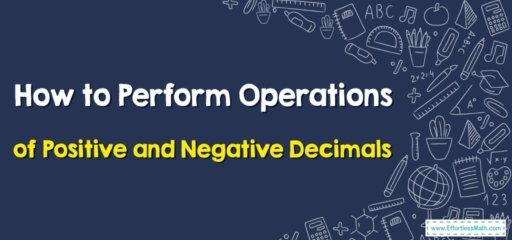# How to Perform Operations of Positive and Negative Decimals

Hello mathematics enthusiasts! In today's lesson, we're going to demystify how to work with positive and negative decimals. This is a fundamental skill that you'll need not only in advanced math courses but also in everyday scenarios. So, let's get started!## Understanding Positive and Negative Decimals

Decimals represent fractions and can either be positive or negative. Positive decimals are greater than zero and located to the right of zero on the number line. Negative decimals are less than zero and are located to the left of zero on the number line.

The operations we can perform on positive and negative decimals include addition, subtraction, multiplication, and division. Remember, working with decimals is just like working with whole numbers, but you need to pay attention to the decimal point and the sign of the number.

## Step-By-Step Guide to Operating Positive and Negative Decimals

### Step 1: Identify the Operation

Determine which operation you need to use. This could be addition, subtraction, multiplication, or division.

### Step 2: Perform the Operation

Next, carry out the operation. Remember to align decimal points for addition and subtraction. For multiplication and division, follow the rules for positive and negative numbers.

Check your answer to ensure it makes sense, both in the context of the problem and mathematically.

### Example:

Let’s work through an example: Subtract $$-0.4$$ from $$1.2$$.

Step 1: Recognize that you’re asked to perform subtraction.

Step 2: Subtract $$-0.4$$ from $$1.2$$. Remember that subtracting a negative is the same as adding a positive, so $$1.2\ – (-0.4) = 1.2 + 0.4 = 1.6$$.

Step 3: Check your answer. The result is greater than $$1.2$$, which makes sense because you’re subtracting a negative decimal.

Remember, the key to mastering operations with positive and negative decimals is practice. So, grab some problems and start practicing. Keep going, you’re doing great!

### What people say about "How to Perform Operations of Positive and Negative Decimals - Effortless Math: We Help Students Learn to LOVE Mathematics"?

No one replied yet.

X
30% OFF

Limited time only!

Save Over 30%

SAVE $5 It was$16.99 now it is \$11.99